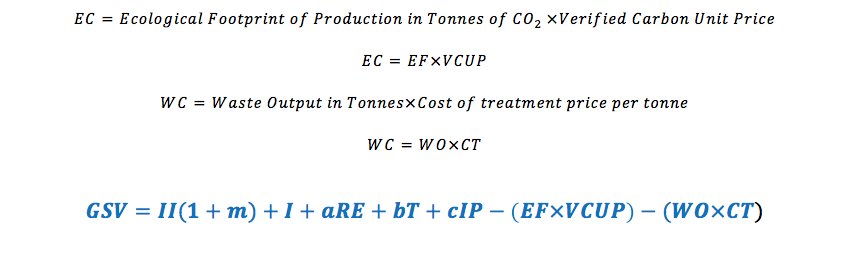# Understanding Time Value of Money and Real Estate Investing

Post on: 3 Апрель, 2016October 16, 2012 By James Kobzeff

It is important for anyone engaged in real estate investing to have a sound working knowledge of the concept of time value of money because it plays a major part in establishing for real estate investors what future cash flows projected from a real estate investment are really worth in todays dollars.

Why is this important? Because the reality is that the purchasing power of money to buy things collected over time becomes less and less as time goes by.

In this article were going to consider the basic components behind time value of money along with an example and illustration. It is not intended to be an exhaustive study on the subject but should give you enough of an idea to understand the role time value plays in real estate investing and why its an important consideration to real estate investors.

#### Components

##### 1. Present Value

The value or worth (today) of a cash flow or series of cash flows that will be available at a specified time or times in the future. In other words, what the expected cash flows from a rental property are worth to the investor in todays dollars relative to purchasing power.

##### 2. Discounting

This is the mathematical procedure for determining present value. In this case, calculations are made on the likely streams of income projected for the future by discounting in order to arrive at the present value of the entire income stream.

The future value or worth (tomorrow) is what a cash flow or series of cash flows will be worth at a specified time in the future.

This is the mathematical procedure for determining future value. When money is placed in an interest-bearing account, for instance, it is compounded by some rate that grows it to a larger amount up to some specified time in the future.

This concerns a series of equal cash flows made at equal time intervals. If an investor collects \$5,000 per month rent from a rental property for the next five months, for instance, it would be an annuity. It would not qualify as such if it were varying amounts of cash or at irregular intervals.

This constitutes a series of uniform cash flows that are made at equal intervals with the payment being made at the beginning of each interval. Rents collected at the beginning of each future month, for instance, would fall in this category.

##### 7. Ordinary Annuity

This constitutes a series of uniform cash flows that are made at equal intervals with the payment being made at the end of each interval. Rents collected at the end of each month, for instance.

The difference in these two types of annuities should be understood to properly grasp the concept of time value. With annuity due, money is collected sooner which means it will have a higher present value as opposed to ordinary annuity which means money is collected more into the future and will have less present value.

This is why real estate investments are studied with consideration for time value of money. For it is highly likely that the timing of cash flows will be more important than the actual amount of cash flows received.

One factor that real estate investors must typically consider is the inflation rate because inflation always erodes purchasing power over time.

To illustrate this well construct a two scenarios in which the real estate investor expects to collect rents of \$60,000 per year for each of the next three years. For the first scenario well assume no inflation rate and therefore the cash flows will not be discounted to arrive at present value. In the second scenario well use an annual inflation rate of 2.7% to discount the cash flows for present value.

##### Scenario 1

Cash flows x years = present value

More from this section
Categories
Tags

• Most Popular